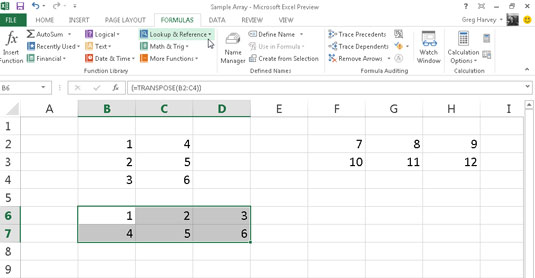##### Excel 2013 All-in-One For DummiesThe TRANSPOSE function in Excel 2013enables you to change the orientation of a cell range (or an array). You can use this function to transpose a vertical cell range where the data runs down the rows of adjacent columns to one where the data runs across the columns of adjacent rows and vice versa.

To successfully use the TRANSPOSE function, not only must you select a range that has an opposite number of columns and rows, but you must also enter it as an array formula.

For example, if you’re using the TRANSPOSE function to transpose a 2 x 5 cell range (that is, a range that takes up two adjacent rows and five adjacent columns), you must select a blank 5 x 2 cell range (that is, a range that takes five adjacent rows and two adjacent columns) in the worksheet before you use the Insert Function button to insert the TRANSPOSE function in the first cell.

Then, after selecting the 2 x 5 cell range that contains the data that you want to transpose in the Array text box of the Function Arguments dialog box, you need to press Ctrl+Shift+Enter to close this dialog box and enter the TRANSPOSE function into the entire selected cell range as an array formula (enclosed in curly braces).

Suppose that you want to transpose the data entered into the cell range A10:C11 (a 2 x 3 array) to the blank cell range E10:F12 (a 3 x 2 array) of the worksheet. When you press Ctrl+Shift+Enter to complete the array formula, after selecting the cell range A10:C11 as the array argument, Excel puts the following array formula in every cell of the range:

`{=TRANSPOSE(A10:C11)}`

The cell range B2:C4 contains a 3 x 2 array in the cell range B2:C4 to a 2 x 3 array in the range B6:D7; follow these steps:

1. Select the blank cell range B6:D7 in the worksheet.

2. Click the Lookup & Reference command button on the Ribbon’s Formulas tab and then choose the TRANSPOSE option from the button’s drop-down menu.

Excel inserts =TRANSPOSE() on the Formula bar and opens the Function Arguments dialog box where the Array argument text box is selected.

3. Drag through the cell range B2:C4 in the worksheet so that the Array argument text box contains B2:C4 and the formula on the Formula bar now reads =TRANSPOSE(B2:C4).

4. Press Ctrl+Shift+Enter to close the Insert Arguments dialog box (don’t click OK) and to insert the TRANSPOSE array formula into the cell range B6:D7 as shown.Clicking the OK button in the Function Arguments dialog box inserts the TRANSPOSE function into the active cell of the current cell selection. Doing this returns the #VALUE! error value to the cell. You must remember to press Ctrl+Shift+Enter to both close the dialog box and put the formula into the entire cell range.

If all you want to do is transpose row and column headings or a simple table of data, you don’t have to go through the rigmarole of creating an array formula using the TRANSPOSE function. Simply copy the range of cells to be transposed with the Copy command button on the Home tab of the Ribbon.

Position the cell cursor in the first empty cell where the transposed range is to be pasted before you click the Transpose option on the Paste command button’s drop-down menu.